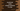# Different index related methods in JavaScript array## JavaScript array methods to find the index of elements:

JavaScript array provides a couple of methods to find the index of an item in it. In this post, we will learn these methods with examples for each. Index starts from 0 in an array and if we get the index, we can read and edit the value in that index for that array.

Mainly, following three methods are used to get the index of an element in an array in JavaScript:

• findIndex()
• indexOf()
• lastIndexOf()

Let’s check each of these methods one by one.

### findIndex():

This method can be used to find the index of the first value in an array that satisfy a given function. This method returns the index of the value if it finds, else it returns -1.

Let’s take a look at the below example:

``````weekdays = ['sun', 'mon', 'tues', 'thurs', 'fri', 'sat'];

console.log(weekdays.findIndex(e => e === 'thurs'));
console.log(weekdays.findIndex(e => e.startsWith('t')));``````

Here,

• The first log statement is finding the index of the first element that is equal to thurs.
• The second log statement is finding the index of the first element that is starting with t.

If you run the above program, it will print the below output:

``````3
2``````

The first log printed the index for thurs, i.e. 3 and the second log printed the index for tues, i.e. 2.

### indexOf:

indexOf takes the element to find in the array. It returns the first index of the element if it finds in the array. Else, it returns -1. For example:

``````weekdays = ['sun', 'mon', 'tues', 'thurs', 'fri', 'sat'];

console.log(weekdays.indexOf('thurs'));
console.log(weekdays.indexOf('hello'));``````

It will print the below output:

``````3
-1``````

Here,

• The first prints the index of thurs in the array weekdays, which is 3.
• The second prints the index of hello in the same array. But, we don’t have this string in this array. So, it returns -1.

Optionally, we can also provide one starting index as the second parameter of indexOf. This is the start index to look for the element in the array.

Let’s take a look at the below program:

``````weekdays = ['sun', 'mon', 'tues', 'thurs', 'fri', 'sat', 'sun'];

console.log(weekdays.indexOf('sun'));
console.log(weekdays.indexOf('sun', 2));``````

Here,

• I added two sun strings in the array weekdays.
• In first log statement, it is finding the index for sun starting from the 0th index. But in the second log statement, it is finding the index for sun starting from the 2nd index. So, for both, it will print different results.

If you run the above program, it will print the below output:

``````0
6``````

For the first one, it printed the index of the first sun. For the second one, it printed the index of the second sun, i.e. the string at 6th index.

Note that, we can also provide a negative value for the start index. If we provide a negative value, it is taken as the offset from the end of the array.

### lastIndexOf:

lastIndexOf is similar to indexOf. The only difference is that lastIndexOf returns the last index of an element in an array. If the element is not found, it returns -1.

It is similar to the indexOf method. It can also take one second index position optionally as the second parameter. If this is given, it will start the searching in backward from that index.

``````weekdays = ['sun', 'mon', 'tues', 'thurs', 'fri', 'sat', 'sun'];

console.log(weekdays.lastIndexOf('sun'));
console.log(weekdays.lastIndexOf('sun', 2));``````

It will give the below output:

``````6
0``````

For the first one, it printed the index of the last occurrence of sun, i.e. 6. For the second log statement, it started the search from 2nd index. Note that, we can also provide a negative value for the start index. If we provide a negative value, it is taken as the offset from the end of the array.